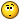# Applying Force/ Impulse to P2 Physics Body on collision

## Recommended Posts

I have a p2 Object with the following properties

``````this.game.physics.p2.enable(this.player, true);
this.player.body.clearShapes();
this.player.body.allowRotation = false;
this.player.body.fixedRotation = true;
this.player.body.immovable = true;
this.player.body.setCollisionGroup(playerCollision);
this.player.body.collides(ballCollision);
this.player.body.kinematic = true;
this.player.body.data.shapes.sensor = false;
this.player.body.collideWorldBounds = true;``````

The above object can only move along its X axis and I want to trigger push/ hit force to its impacting object on collision

``````this.game.physics.p2.enable(this.ball);
this.ball.body.setCircle(28);
this.ball.body.collideWorldBounds = true;
this.ball.body.allowRotation = true;
this.ball.body.fixedRotation = false;
this.ball.body.setCollisionGroup(ballCollision);
this.ball.body.collides(playerCollision);``````

The above object is always the impacting object whose movement is controlled by gravity and impulse.

I'm trying to figure out how to calculate the following:-

• The point of collision on the body of "this.player"
• The impulse to apply to the impacting object after collision to simulate a proper/ accurate deflection setting the projected angle and speed/ velocity
• what properties of the impacting object do I set? Force? AngularVelocity? Velocity?

##### Share on other sites

Ok

Been researching and tinkering around and came up with this

``````this.player.body.onBeginContact.add(function(body, bodyBody, shapeA, shapeB, equation )
{

let mass = .53 // mass of a fist punch
let duration = 2; // default 2 ms
let localX = equation.contactPointB;
let localY = equation.contactPointB;
if(this.spaceKey.isDown) // when the user press punch button
{
duration = this.spaceKey.duration; // length of time/ power
}

let impulse = [	(mass * this.player.body.velocity.x) * duration,
((mass * 2) * duration) * -1 // using a static velocity of 2 for a "punch" multiply buy the time the user stays pressing the key
];
body.applyImpulse(impulse,localX,localY); // give the object momentum change
}, this);``````

Not sure if the properties used are correct but I'm getting closer. Not sure of the x and y properties to be using though...is it the punch object or the ball contact point

Not sure why code format is breaking though